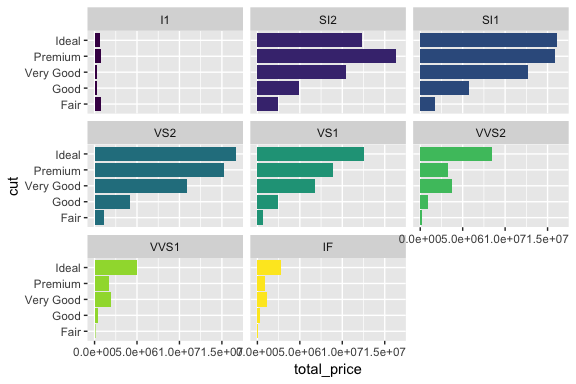# Introduction

Owing to its extensive functionality and its roots in a robust grammar of graphics (Wilkinson 2013), ggplot2 (Wickham 2016) has become very popular among R users. In using ggplot, the general approach to generating plots is to first conceive of the plot and then use our undertsanding of ggolot to create the required mappings, layers and other adjustments. The process requires us to divide our cognitive resources to the main task at hand – extracting intelligence from the data – and the process of generating the requisite plot. The guiding insight behind ezEDA is that the process of exploratory data analysis can be made more productive by increasing the proportion of congitive resources devoted to imagining the kinds of analysis we could perform (by reducing the proportion devoted to the mechanices of generating the plot). Where possible, devoting more of our mental energies to thinking about the kinds of explorations we would like to perform will likely make us more productive.

Although exploratory analysis of data differs from dataset to dataset, we can still see some recurrent themes or patterns that apply to many situations. ezEDA identifies such common patterns, and for each one, it provides a single convenience function that relieves us of the mechanices of generating the plot. With ezEDA we aim to ease the task of generating ggplot-based visualizations by allowing users to think in terms of their problem domain rather than the details of how to achieve the plot that they have in mind. This approach is particularly useful when the visualization task involves standard themes or patterns. The ezEDA package currently provides functions for twelve patterns and we aim to incorporate more in future versions.

ezEDA is only beneficial in situations where the analyst is able to exploit a common pattern that ezEDA has already identified. For other situations, the analyst has to use other means like constructing a ggplot plot from the ground up. Here are some general features of ezEDA functions:

• all ezEDA functions are built on top of ggplot functions
• like dplyr and ggplot, ezEDA functions make interactive use easier by allowing the use of unquoted column names and support variable numbers of arguments
• given that the main focus of ezEDA functions is to enable users to quickly generate visualizations for supported patterns, these functions support limited customization; they do not allow the full range of customization that a direct use of ggplot would

Once they have completed their initial explorations and obtained the necessary insights, it could very well be that users will then dive into ggplot and fine-tune their plots where needed.

Currently, ezEDA provides functions under five categories:

• Trends
• Distributions
• Relationships
• Tallies, and
• Contributions

# Basic concepts behind design of the ezEDA interface

ezEDA is partly motivated by the work of Stephen Few (Few 2015) who described a general framework for Exploratory Data Analysis. Such a framework would be useful when we are presented with a dataset and would like to generate questions to ask of the dataset – questions that could potentially generate interesting results or confirm what we already knew or suspected. Few’s framework is driven by the observation that any dataset has columns of four types:

• categories – categorical columns representing categories with no inherent numerical meaning
• measures – numerical columns
• time – columns representing time (could be date columns or just integers representing sequential time units)
• others – columns without much significance for data exploration (like names, etc.)

Once we establish this terminology, the list below shows the patterns and the corresponding ezEDA functions:

• trends of measures
• functions: measure_change_over_time_wide and measure_change_over_time_long
• distributions of measures
• functions: measure_distribution, measure_distribution_by_category, measure_distribution_by_two_categories and measure_distribution_over_time
• relationships between measures
• functions: two_measure_relationship and multi_measure_relationship
• tallies for counts of one or two categories
• functions: category_tally and two_category_tally
• contributions of different categories to a measure
• functions: category_contribution and two_category_contribution

ezEDA provides functions for these tasks. The package currently has twelve functions and we hope to add more as we identify more patterns.

# Installing ezEDA

ezEDA is available under CRAN and can be installed as usual by calling the install.packages function:

install.packages("ezEDA")

ezEDA depends on many other packages like ggplot2, dplyr, tidyr and others and if any of those packages are not installed on your system, the above code will cause any of the missing packages to be installed as well. # Using ezEDA As always, it is a good idea to make the package namespace available via the library function:

library(ezEDA)

# ezEDA functions

We discuss each of the functions below. For convenience, we have divided the functions into convenient groups.

## Distributions

Very often we study the distributuons of numeric columns (measures).ezEDA provides four different functions. Within this broad theme, we are often also interested in studying how the distribution changes based on one or two categories or based on time.

### measure_distribution

Distribution of a measure: default is histogram.

## For ggplot2::mpg, plot the distribution of highway mileage
measure_distribution(ggplot2::mpg, hwy)
#> Using binwidth corresponding to 30 bins. Use bwidth argument to fix if needed.
#> stat_bin() using bins = 30. Pick better value with binwidth.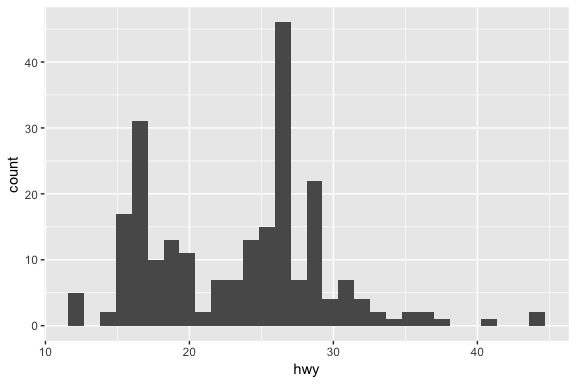Distribution of a measure: default is histogram. By default the function uses a bin width corresponding to 30 bins. Use the bwidth argument to specify the desired value for the width of each bin.

## For ggplot2::mpg, plot the distribution of highway mileage
measure_distribution(ggplot2::mpg, hwy, bwidth = 2)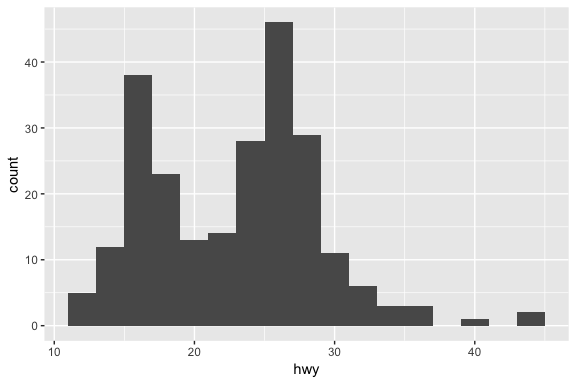Distribution of a measure. Get a boxplot instead of histogram.

## For ggplot2::mpg, plot the distribution of highway mileage as a boxplot
measure_distribution(ggplot2::mpg, hwy, type = "box")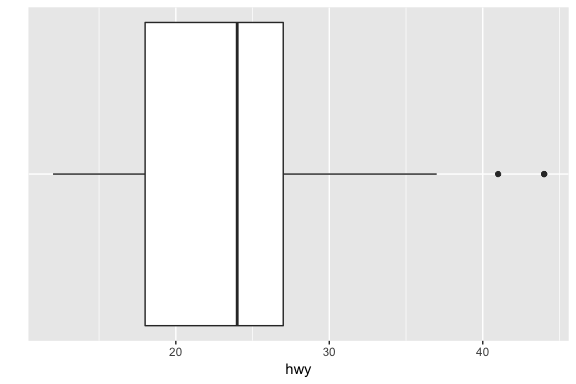### measure_distribution_by_category

Distribution of a measure with highlighting of different values of a single category.

## For ggplot2::diamonds, plot the distribution of price while highlighting
## the counts of diamonds of different cuts
measure_distribution_by_category(ggplot2::diamonds, price, cut)
#> Using binwidth corresponding to 30 bins. Use bwidth argument to fix if needed.
#> stat_bin() using bins = 30. Pick better value with binwidth.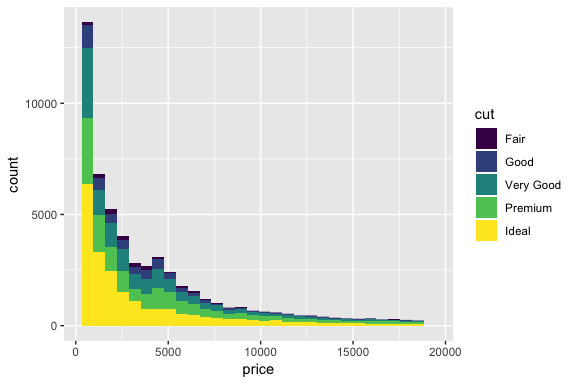Distribution of a measure with highlighting of different values of a single category across facets.

## For ggplot2::diamonds, plot the distribution of price showing
## the distribution for each kind of cut in a different facet
measure_distribution_by_category(ggplot2::diamonds, price, cut, separate = TRUE)
#> Using binwidth corresponding to 30 bins. Use bwidth argument to fix if needed.
#> stat_bin() using bins = 30. Pick better value with binwidth.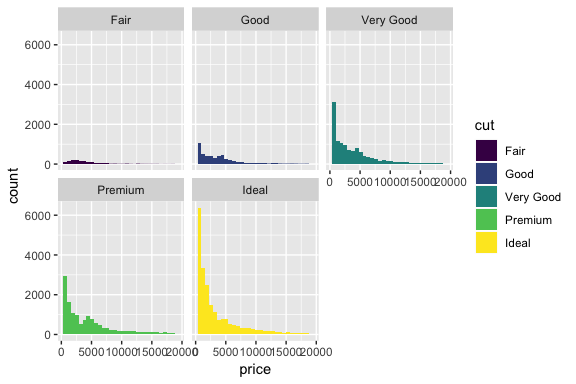### measure_distribution_by_two_categories

Distribution of a measure for a combination of two categories within a facet grid

## For ggplot2::diamonds, plot the distribution of price separately
## for each unique combination of cut and clarity
measure_distribution_by_two_categories(ggplot2::diamonds, carat, cut, clarity)
#> Using binwidth corresponding to 30 bins. Use bwidth argument to fix if needed.
#> stat_bin() using bins = 30. Pick better value with binwidth.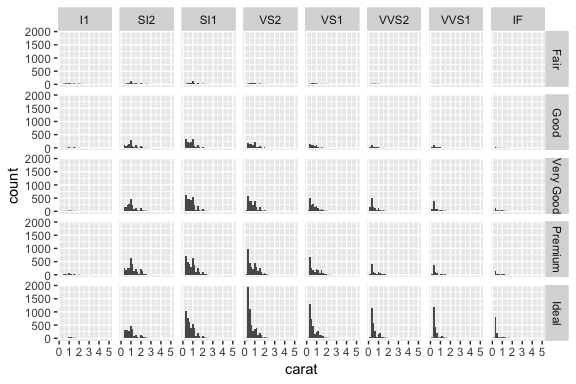### measure_distribution_over_time

Study the change of distribution of a measure over time

## 50 random values of three measures for each of 1999, 2000 and 2001
h1 <- round(rnorm(50, 60, 8), 0)
h2 <- round(rnorm(50, 65, 8), 0)
h3 <- round(rnorm(50, 70, 8), 0)
h <- c(h1, h2, h3)
y <- c(rep(1999, 50), rep(2000, 50), rep(2001, 50))
df <- data.frame(height = h, year = y)
measure_distribution_over_time(df, h, year)
#> Using binwidth corresponding to 30 bins. Use bwidth argument to fix if needed.
#> stat_bin() using bins = 30. Pick better value with binwidth.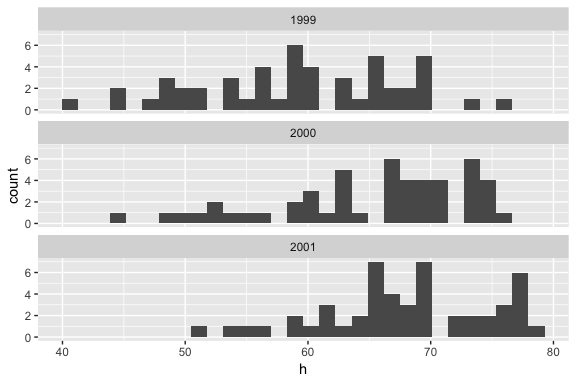## Relationships

WHen a dataset has two or more measures, studying their pairwise relationships often yields useful insights. exEDA provides two relevant functions.

### two_measures_relationship

Scatterplot of the relationship between two measures with optional coloring of points by a category.

## For ggplot2::mpg, plot the highway mileage against the displacement
two_measures_relationship(ggplot2::mpg, displ, hwy)
#> geom_smooth() using method = 'loess' and formula 'y ~ x'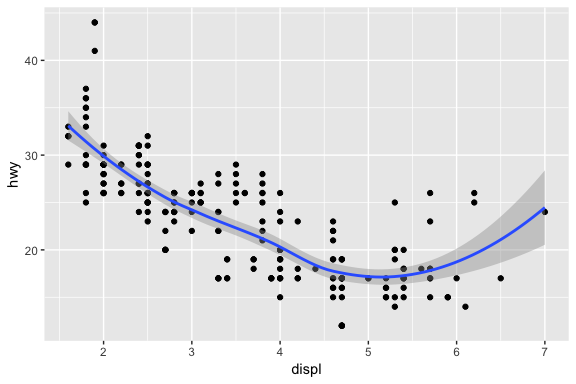Below is a variant shopwing the relationship separately for each value of a category.

## For ggplot2::diamonds, plot the price against carat
## while showing the relationship separately for diamionds of
## each kind of cut
two_measures_relationship(ggplot2::diamonds, carat, price, cut)
#> geom_smooth() using method = 'gam' and formula 'y ~ s(x, bs = "cs")'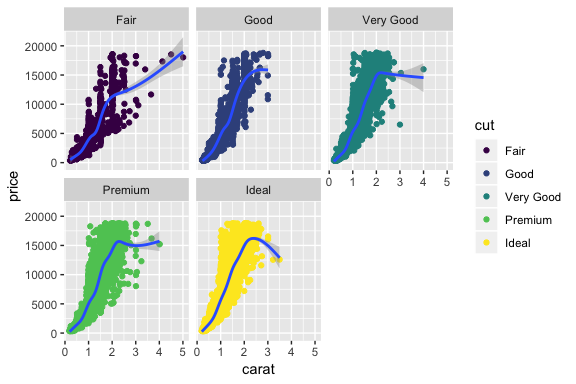### multi_measures_relationship

Scatterplot matrix showing the relationships between three or more measures.

## For ggplot2::mpg, plot the relationship between city mileage,
## highway mileage and displacement
multi_measures_relationship(ggplot2::mpg, cty, hwy, displ)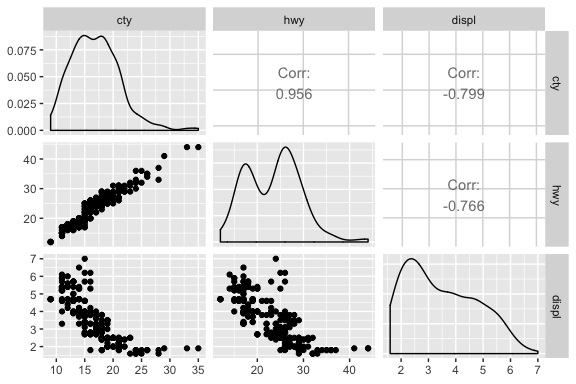## Tallies

The two functions in this group satisfy a common need to generate counts of categories. For example, in a dataset of students, we might want to plot the counts of students by gender; in the diamonds dataset from ggplot2, we might want to generate a plot of the number of diamonds by color, and so on.

### category_tally

Barplot of the counts based on a category column. Often when a barplot has many bars, we run the risk of the x-axis labels running onto each other. To handle this issue, the function flips the coordinates. The first argument is the dataset to be used and the second argument is the unquoted name of the relevant category column.

## For ggplot2::mpg, plot the tallies for the different vehicle classes
category_tally(ggplot2::mpg, class)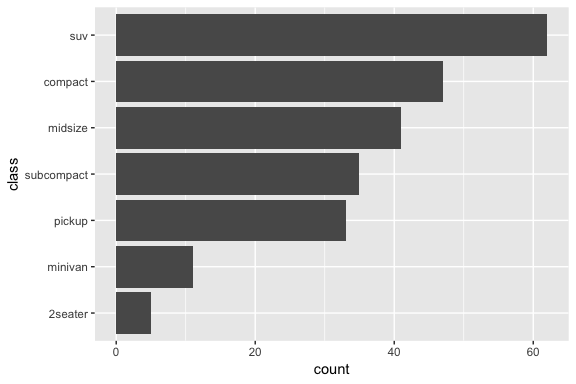### two_category_tally

Barplot of one category showing its conposition in terms of another category. The arguments are: the dataset to be used and the unquoted names of the two category columns.

## For ggplot2::diamonds plot the tallies for different types of
## cut and clarity
two_category_tally(ggplot2::diamonds, cut, clarity)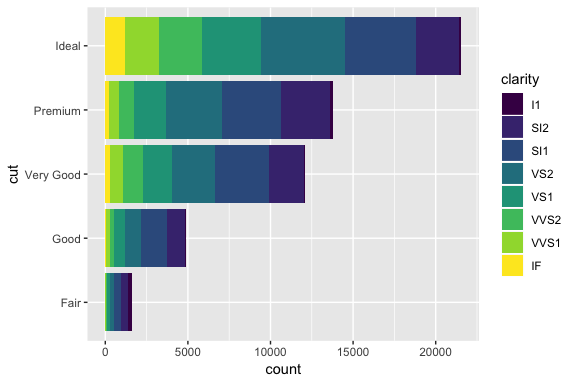Below is a variant with facets.

## For ggplot2::diamonds, plot the tallies for different types of
## cut and clarity and show the plots for each value of the
## second category in a separate facet
two_category_tally(ggplot2::diamonds, cut, clarity, separate = TRUE)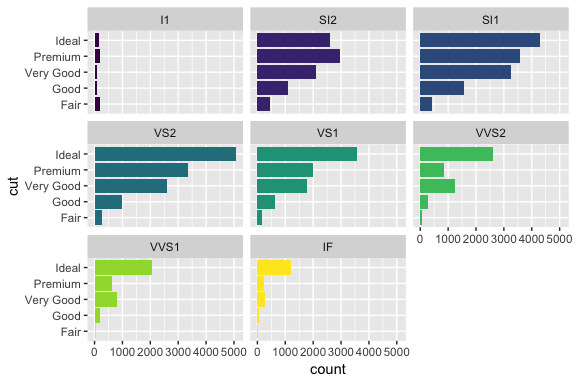## Contributions

The two functions in this group satisfy a common need to analyze the extent to which specific categories contribute to specific measures. For example, in a dataset of people, we might want to plot the total income by gender; in the diamonds dataset from ggplot2, we might want to generate a plot of the contribution to the price by diamonds of various kinds of cut, and so on.

### category_contribution

Barplot of the total of a measure based on a category column.

## For ggplot2::diamonds, plot the total price for each kind of cut
category_contribution(ggplot2::diamonds, cut, price)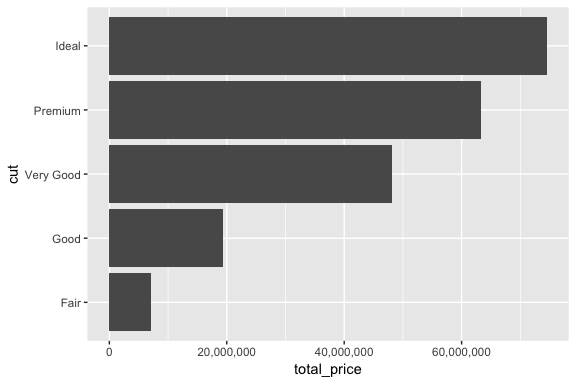### two_category_contribution

Barplot of the total of a measure based on a category column.

## For ggplot2::diamonds, plot the total price for each kind of cut
## while also showing the contribution of each kind of clarity
## within each kind of cut
two_category_contribution(ggplot2::diamonds, cut, clarity, price)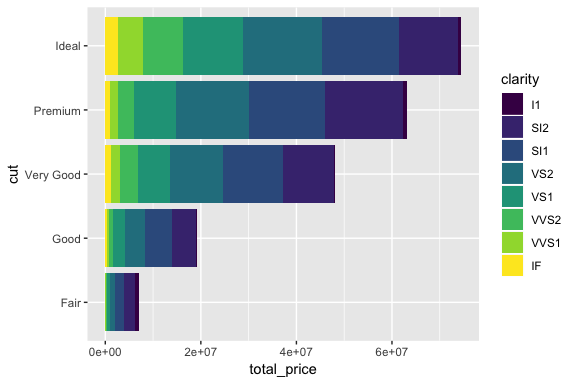Below is a variant with facets.

## For ggplot2::diamonds, same as above, but show each color
## on a different facet
two_category_contribution(ggplot2::diamonds, cut, clarity, price, separate = TRUE)